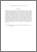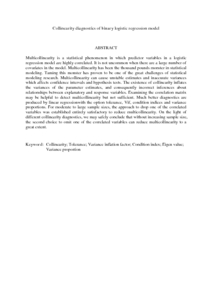# Collinearity diagnostics of binary logistic regression model

## Citation

Midi, Habshah and Sarkar, Saroje Kumar and Rana, Sohel (2010) Collinearity diagnostics of binary logistic regression model. Journal of Interdisciplinary Mathematics, 13 (3). pp. 253-267. ISSN 0972-0502; ESSN: 2169-012X

## Abstract

Multicollinearity is a statistical phenomenon in which predictor variables in a logistic regression model are highly correlated. It is not uncommon when there are a large number of covariates in the model. Multicollinearity has been the thousand pounds monster in statistical modeling. Taming this monster has proven to be one of the great challenges of statistical modeling research. Multicollinearity can cause unstable estimates and inaccurate variances which affects confidence intervals and hypothesis tests. The existence of collinearity inflates the variances of the parameter estimates, and consequently incorrect inferences about relationships between explanatory and response variables. Examining the correlation matrix may be helpful to detect multicollinearity but not sufficient. Much better diagnostics are produced by linear regressionwith the option tolerance, Vif, condition indices and variance proportions. For moderate to large sample sizes, the approach to drop one of the correlated variables was established entirely satisfactory to reduce multicollinearity. On the light of different collinearity diagnostics, we may safely conclude that without increasing sample size, the second choice to omit one of the correlated variables can reduce multicollinearity to a great extent.Preview
PDF (Abstract)
Collinearity diagnostics of binary logistic regression model.pdfView Item